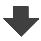$\definecolor{red}{RGB}{255,0,0}$ $\definecolor{black}{RGB}{0,0,0}$ $\definecolor{blue}{RGB}{0,38,255}$Search:

# Chemical thermodynamics

## Calorimetry

### Exercise 1

$1.000\;kg$ water at $0^oC$ are mixed with $0.100\;kg$ water at $90^oC$. Neglecting the heat exchange with the container, it is asked to calculate the final temperature.

The energy is conserved: Heat received by the cold water = Heat lost by the warm water Let be $x$ the final temperature: $4184\cdot 1\cdot (x-0)$ $=$ $\color{red}-\color{black}4184\cdot 0,1\cdot (x-90)$ $x \approx 8.2^oC$